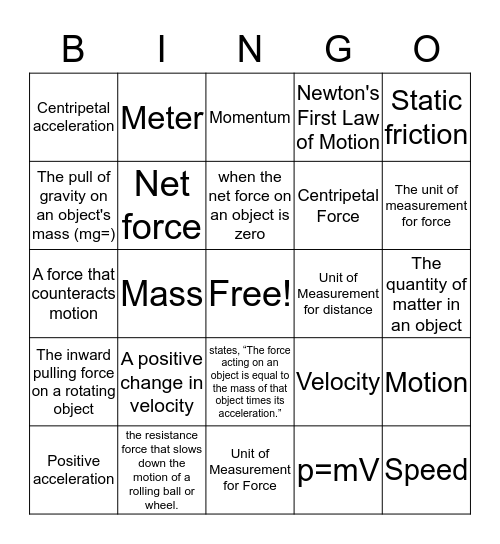# PHYSICS BINGOThis bingo card has a free space and 57 words: Acceleration, Positive acceleration, Negative acceleration, Centripetal acceleration, Unit of Measurement for Force, Force, Net force, Equilibrium /Balanced, Centripetal Force, Newton, Newton's First Law of Motion, Inertia, Newton's Second Law of Motion, Newton's Third Law of Motion, Friction, Rolling friction, Static friction, Mass, Weight, Speed, Unit of Measurement for distance, V = d/t, Velocity, Momentum, A push or a pull, when the net force on an object is zero, An object at rest remains at rest; and if in motion will remain in motion in a straight line; unless a force acts on it, This acts on a mass to create the force of weight, The unit of measurement for force, The sum of all forces acting on an object, An objects resistance to change its motion, The rate at which velocity is changing, distance traveled divided by time taken, speed + direction, The quantity of matter in an object, The pull of gravity on an object's mass (mg=), An objects resistance to change in its motion, A force that counteracts motion, P = mV, The inward pulling force on a rotating object, Meter, states, “The force acting on an object is equal to the mass of that object times its acceleration.”, Momentum, The unit of measurement for forces, Orbit, A negative change in velocity, A positive change in velocity, the resistance force that slows down the motion of a rolling ball or wheel., the frictional force that prevents two surfaces from sliding past each other, states that for every action, there is an equal and opposite reaction., also known as the law of inertia, p=mV, Slope, Rise over run, the gravitationally curved path of an object around a point in space, Motion and a change in position of an object.

⚠ This card has duplicate items: Momentum (2)

## Play Online